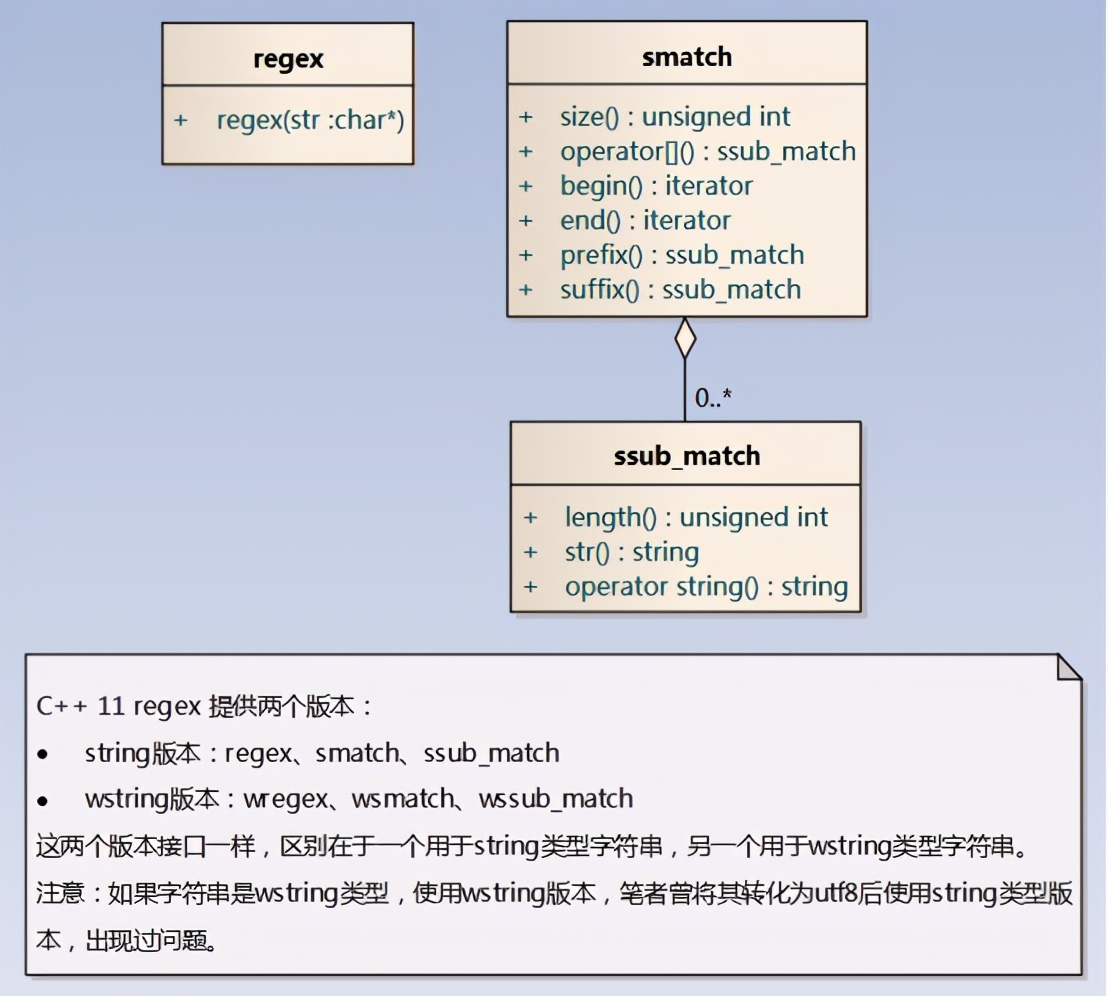# 正则表达式匹配指定字符串(正则表达式使用实例)

C++11标准支持正则表达式后，使用正则表达式查找、提取、替换字符串就无需使用第三方开源库。类regex，用来存放正则表达式，其实就是一个字符串，只需关心构造函数，其需要一个字符串作为参数进行对象构造。正则表达式语法遵循ECMAScript标准。类smatch，用来存放查找、提取操作的结果，其实就是一个ssub_match的数组，正则表达式语法支持使用括号来获得某个子匹配，所以匹配结果会有多个，第

C++11标准支持正则表达式后，使用正则表达式查找、提取、替换字符串就无需使用第三方开源库。类regex，用来存放正则表达式，其实就是一个字符串，只需关心构造函数，其需要一个字符串作为参数进行对象构造。正则表达式语法遵循ECMAScript标准。类smatch，用来存放查找、提取操作的结果，其实就是一个ssub_match的数组，正则表达式语法支持使用括号来获得某个子匹配，所以匹配结果会有多个，第一个存完整匹配结果，其它存正则表达式指定的子匹配。比如字符串 subsentence，对于正则表达式sub来说，regex_match()是失败的，因为尾部sentence没有被匹配到，regex_search()是成功的。下面代码示例演示如何使用C++11的regex API，理解透该示例代码，你就基本掌握了其使用方法。

C++11标准支持正则表达式后，使用正则表达式查找、提取、替换字符串就无需使用第三方开源库。``1、bool regex_search(string s, regex e);2、bool regex_search(string s, smatch m, regex e);``

``string regex_replace(string s, regex e, string fmt);``

``#include <string>#include <regex>#include <iostream> int main(){    const std::string s = "this subject has a submarine as a subsequence";    const std::regex e("\\b(sub)([^ ]*)");  // sub开头的单词     // 只要查找是否有sub开头的单词    if (std::regex_search(s, e))    {        std::cout << "the source string contains word beginning by sub" << std::endl;    }     // 查找所有sub开头的单词，并打印出来    std::smatch m;  //存放查找结果    std::string s2 = s;    while (std::regex_search(s2, m, e))    {        for (auto x : m)  // 正则表达式有两个括号，m共有3个元素        {            std::cout << x << " ";        }        std::cout << std::endl;        s2 = m.suffix();  // 指向查找结果的下一个位置，继续查找    }     // 只要判断是否匹配    if (!std::regex_match(s, e))  // 要求完全匹配，这点跟查找不同    {        std::cout << "the source string is not match" << std::endl;    }     // 如果匹配，输出匹配结果    std::regex e2("(.*)sub(.*)");  // 含有关键词sub，并提取sub前和sub后的内容    if (std::regex_match(s, m, e2)) // 匹配成功了，m对象才是有效存放提取内容    {        for (unsigned i = 1; i < m.size(); i++)  // 第1个元素就是s，这里就不打印        {            // 注意：因为正则表达式.*是尽可能的去匹配，所以关键词sub匹配的是            // 最后一个单词subsequence的sub            std::cout << m[i] << std::endl;          }    }     // 把subsequence替换成sub-sequence    std::regex e3("subsequence");        std::cout << std::regex_replace(s, e3, "sub-sequence") << std::endl;     // 把所有sub开头的单词，在sub后面加-    // \$2匹配结果smatch中第2个元素，也就是sub后面的内容    std::cout << std::regex_replace(s, e, "sub_\$2") << std::endl;}``